# Data Type cf for Correlation Functions

hadron provides a data type or better class for correlation functions and correlation matrices, which is called cf. There is a whole list of input routines available to import data from HDF5, text or binary formats into the cf container. The most important ones are compiled in the following table:

Hadron Function Correlator Format
readtextcf text
readbinarycf binary, HDF5
readbinarysamples binary
more?

For even more flexibility there is the raw_cf container, for which we refer to the documentation.

In oder to solve the generalised eigenvalue problem (GEVP) one has to read several correlation functions into one cf correlator matrix. For this purpose the combine operation c is defined for the class cf. Thus, for instance the following code snipped can be used:

Time <- 48
correlatormatrix <- cf()
for(i in c(1:4)) {
tmp <- readbinarycf(files=paste0("corr", i, ".dat"), T=Time)
correlatormatrix <- c(correlatormatrix, tmp)
}
rm(tmp)


This code snippet reads a correlator matrix with four correlation functions from four files. The read functions can also directly read from a list of files. File lists can be created conveniently using the following routines

getorderedfilelist <- function(path="./", basename="onlinemeas",
last.digits=4, ending="")
getconfignumbers <- function(ofiles, basename="onlinemeas",
last.digits=4, ending="")
getorderedconfigindices <- function(path="./", basename="onlinemeas",
last.digits=4, ending="")


for which we refer to the documentation.

# Resampling Strategies

Once the bare data is available as a cf, one has to decide for an error analysis strategy. This can be either the bootstrap or the jackknife. To demonstrate this we first load the sample correlation matrix provided by hadron

data(correlatormatrix)


which corresponds to a $$2\times 2$$ local-fuzzed correlator matrix with quantum numbers of the pion. First the resampling needs to be performed, for instance for the (blocked) bootstrap

boot.R <- 150
boot.l <- 1
seed <- 1433567
correlatormatrix <- bootstrap.cf(cf=correlatormatrix,
boot.R=boot.R,
boot.l=boot.l,
seed=seed)


Analogously, jackknife.cf initiates the jackknife resampling. boot.R is the number of bootstrap replicates, boot.l the block lentgh. Now, it is also possible to plot the data with errors

plot(correlatormatrix, log="y",
xlab=c("t/a"), ylab="C(t)")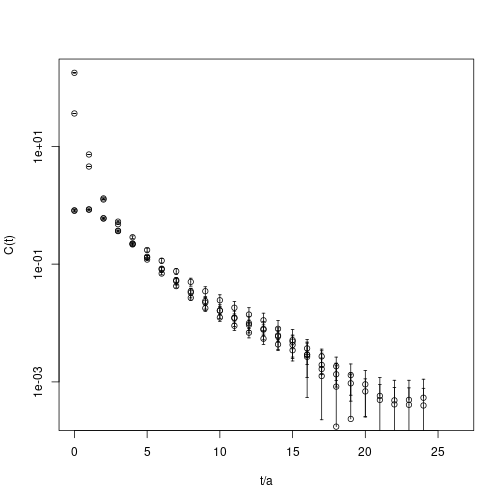# Solving the GEVP

Let us denote the correlator matrix by $$C(t)$$. Now we are going to solve the generalised eigenvalue problem $C(t)\, v_i(t, t_0)\ =\ \lambda_i(t, t_0)\, C(t_0)\, v_i(t, t_0)$ with some reference time value $$t_0$$. One can show that the so-called principal correlators $$\lambda(t, t_0)$$ follow for large $$t$$-values the following behaviour $\lambda_i(t, t_0)\ \propto\ e^{-E_i(t-t_0)} + e^{-E_i(T-t+t_0)}\,.$ Here, $$T$$ is the time extent and we focus on a symmetric correlation matrix in time. However, analogously one can show this with a minus sign for anti-symmetric correlation matrices in time. Of course, we also have $$\lambda(t_0, t_0) = 1$$. We re-write the generalised eigenvalue problem by defining $w_i\ =\ \sqrt{C(t_0)} v_i$ and solve the simple eigenvalue problem $\sqrt{C(t_0)}^{-1}\,C(t)\,\sqrt{C(t_0)}^{-1}\, w_i\ =\ A\, w_i\ =\ \lambda_i(t, t_0)\, w_i$ instead.

In hadron this task is performed as follows on the bootstrap correlator matrix in the most simple case

t0 <- 4
correlatormatrix.gevp <- bootstrap.gevp(cf=correlatormatrix, t0=t0,
element.order=c(1,2,3,4),
sort.type="values")


Next, the principal correlators $$\lambda_i$$ are obtained as follows, where in this case we have $$i=1,2$$

pc1 <- gevp2cf(gevp=correlatormatrix.gevp, id=1)
pc2 <- gevp2cf(gevp=correlatormatrix.gevp, id=2)
plot(pc1, col="red", pch=21, log="y", xlab="t", ylab="C(t)")
plot(pc2, rep=TRUE, col="blue", pch=22)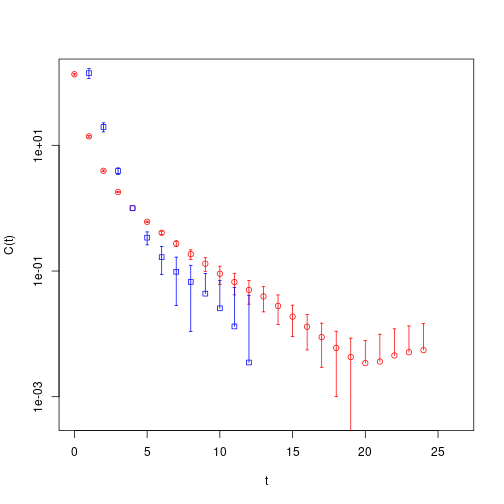These principal correlators can be analysed as every object of type cf, see below.

## Additional Options

bootstrap.gevp has some additional options which are worth mentioning.

1. During the bootstrap procedure for the GEVP, eigenvalues have to be sorted for every $$t$$-value. This can be either done by values, vectors or det passed via the parameter sort.type. When vectors is chosen, scalar products of eigenvectors are computed $v(t', t_0) \cdot v(t, t_0)$ and the overlap maximised. When sort.t0 is set to TRUE, the comparison time is chosen constant as $$t'=t_0+1$$. Otherwise, $$t'=t-1$$ is set in dependence of $$t$$.

2. With parameter element.order the correlation functions in the input correlator matrix are specified for use in the GEVP. This can be a sub-set of all the correlation functions in the matrix. Double usage is allowed as well.

# Extracting Energies

## Matrixfit

First, a fit directly to the (principal) correlator can be performed. The corresponding functionality is provided in hadron by the function matrixfit and, more modern, new_matrixfit. Let us discuss here the former in its application to

pc1.matrixfit <- matrixfit(cf=pc1, t1=6, t2=21, useCov=TRUE,
parlist=array(c(1,1), dim=c(2,1)),
sym.vec=c("cosh"), fit.method="lm")

## Loading required namespace: minpack.lm

plot(pc1.matrixfit, do.qqplot=FALSE,
xlab="t", ylab="C(t)")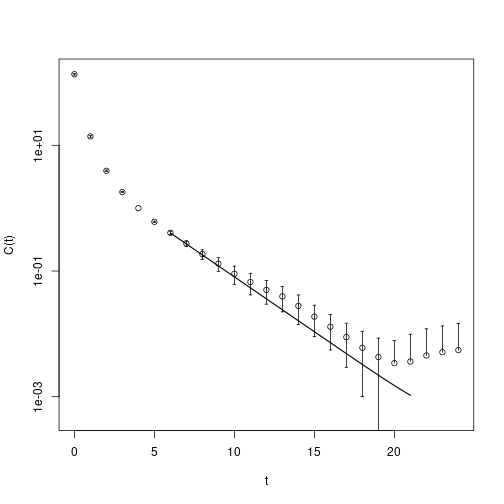An extended overview is provided by the overloaded summary function

summary(pc1.matrixfit)

##
##  ** Result of one state exponential fit **
##
## based on 541 measurements
## time range from 6  to  21
##
## ground state energy:
## E    =    0.4020216
## dE   =    0.05814395
##
## Amplitudes:
## P 1  =    2.997469
## dP 1     =    0.4713082
##
## boot.R   =    150  (bootstrap samples)
## boot.l   =    1  (block length)
## useCov   =    TRUE
## chisqr   =    4.182661
## dof  =    14
## chisqr/dof=   0.2987615
## Quality of the fit (p-value): 0.9942633


This yields an energy level with error of $$E =0.402(58)$$.

As we know that $$\lambda(t_0, t_0)=1$$, we can fit more than a single exponential to the principal correlator. For this matrixfit knows the model pc. The corresponding fit model reads $f(t; E, \Delta E, A)\ =\ \exp(-E(t-t_0))(A + (1-A)\exp(-\Delta E(t-t_0))$ involving three fit parameters. Of course, the fit must be started at earlier time slices in order to be sensitive to excited states.

pc1.matrixfit <- matrixfit(cf=pc1, t1=3, t2=20, useCov=TRUE,
parlist=array(c(1,1), dim=c(2,1)),
sym.vec=c("cosh"), fit.method="lm",
model="pc")
plot(pc1.matrixfit, do.qqplot=FALSE,
xlab="t", ylab="C(t)")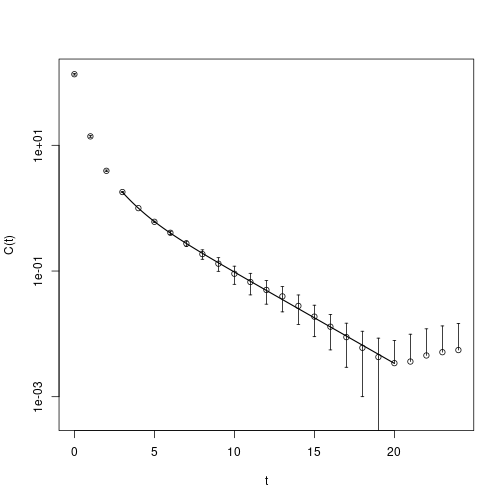A useful crosscheck is to not plot the raw correlator, but the correlator with the leading exponential divided out

plot(pc1.matrixfit, do.qqplot=FALSE,
xlab="t", ylab="C(t)", plot.raw=FALSE)
abline(h=1, lty=2)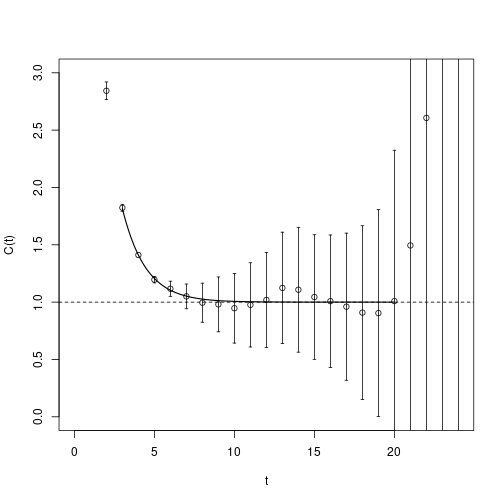In such a plot all the data points should fluctuate around one. This matrixfit gives as a result $$E =0.334(60)$$.

## Effective Masses

Similaryly, effective masses $M_\mathrm{eff}\ =\ -\log\frac{C(t)}{C(t+1)}$ can be computed and bootstrapped as follows

pc1.effectivemass <- fit.effectivemass(cf=bootstrap.effectivemass(cf=pc1),
t1=5, t2=20)
plot(pc1.effectivemass, col="red", pch=21, ylim=c(0,1),
xlab="t", ylab="Meff")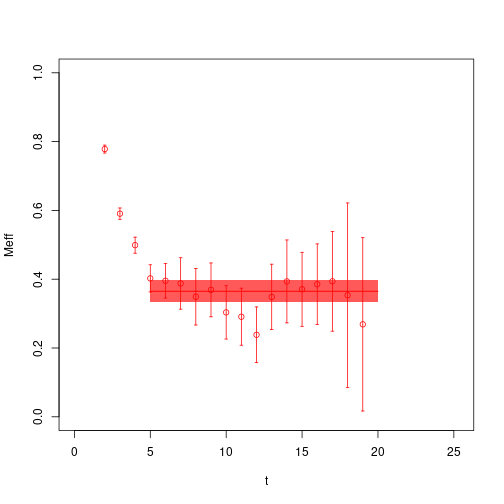From the fit to the effective masses we obtain in this case $$E =0.365(33)$$.